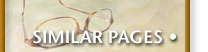## Node.js Modules

Node.js has a very coherent approach to structuring code. I didn't realize until I was "into" my first project that Node modules follow the Singleton Pattern. Since this wasn't obvious from the Node.js documentation, I thought I would explain it.

Suppose `a.js` wants to call a function in `b.js`, you can write something like:

``````var b = require('b');  // NB: .js is optional
b.callIt();
``````

Unlike JavaScript on the client, variables in Node do not have global scope. This means that `b.js` has to export the functions that `a.js` could call:

``````function someFunction() {
// &hellip; some code &hellip;
}
exports.callIt = someFunction;
``````

You could also make this a bit smaller:

``````exports.callIt = someFunction() {
// &hellip; some code &hellip;
};
``````

However, I don't care much for this optimization since most JavaScript IDEs do not show the function in the Code Outline panel. The other advantage of the first approach is that you can give your functions descriptive names that may be different from how the caller would use them.

## Module Scope

First time some code `require`s a module, it is executed. When another piece of code `require`s that same module, it isn't re-run. While this is efficient, it also means that only one module instance exists in the application.

Why is this important?

Consider the following code:

``````var counter = 0;

function getID() {
return ++counter;
}
exports.id = getID;
``````

The variable, `counter`, is in the module's scope meaning that other functions in the module can access it, but since it isn't exported, no one else can.

But since only one module instance is available per application, this is effectively the Singleton Pattern.

## Advantage of this Singleton Pattern

Some of my modules may require a lot of configuration or initialization. No problem. You can just write that could outside of a function, and it is executed once.

Normally, I create and call an `init` function:

``````function init() {
// Lots of setup work here.
}

init(); // Initialize the module
``````

## Disadvantage of this Singleton Pattern

All variables inside a module are essentially shared with the rest of your application (hidden, but still shared). This may not be what you want. In this case, you need create a JavaScript class:

``````function Foobar(x, y, z) {
this.x = x;
this.y = y;
this.z = z;

this.sum = function() {
return this.x + this.y + this.z;
};
}

exports.getFoobar = function(a, b, c) {
return new Foobar(a, b, c);
};
``````

The `require` function in Node can be chained, which is useful when you only need one function out of it.

``````var foo = require('foobar').getFoobar(1,3,7);
``````
Tell others about this article: